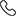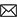Sales :9214233303 / Tech. Support Desk :9214233360/40 (9AM ~ 6PM)# Logarithms, Trigonometry, Quadratic Equations by MC Sir

Logarithms
The logarithm of a number is the exponent by which another fixed value, the base, has to be raised to produce that number.The present-day notion of logarithms comes from renowned mathematician Leonhard Euler, who connected them to the exponential function in the 18th century.

Trigonometry
The study of angles and of the angular relationships of planar and three-dimensional figures is known as trigonometry. The trigonometric functions (also called the circular functions) comprising trigonometry are the cosecant, cosine, cotangent, secant, sine, and tangent.

In mathematics, a quadratic equation is a univariate polynomial equation of the second degree. A general quadratic equation can be written in the form ax^2+bx+c=0 where a,b,c are real numbers and a is not equal to zero.

In this video lecture series, MC Sir wants to focus on these three basic topics as they find extensive application in almost all other chapters of mathematics. From an IIT-JEE perspective, conceptual clarity in these topics is necessary for success in exams.

• Rs.2,800 Rs.2,800
• Rs.2,800 Rs.2,800
• Rs.3,800 Rs.3,800
Subject Medium Target Language Mathematics English XI,XII,XIII Class English + Hindi Topic Based 23 Videos 0 Videos N/A Logarithms, Trigonometry, Quadratic Equations Must for class 11th studying students who want to do well in JEE Main & Advanced Exams. Ideal for class 12th students who are preparing for JEE Main & Advanced Exams. Essential for JEE Main & Advance Exam preparations.

### Other Courses by Manoj Chauhan SirTap a Star to Rate us:
close
close